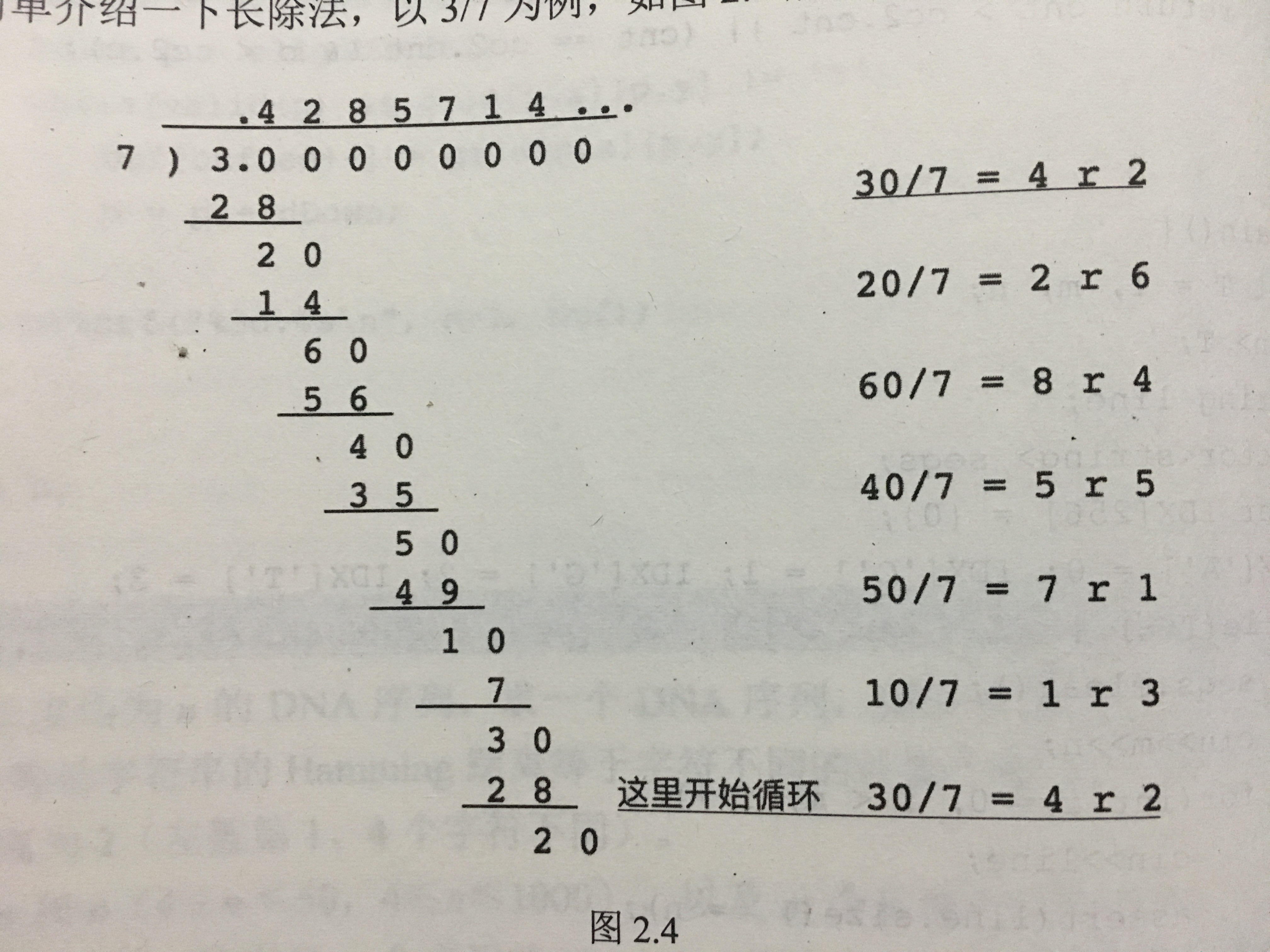# 求循环小数的表示以及循环节长度

①mod = a%b；

②小数 = （mod*10） / b；

③mod = （mod*10）%b；1.  mod = 3%7 = 3；

2. mod = （3*10）/ 7 = 4  （小数点后第一个数）

3. mod = （3*10）%7 = 2

4. mod = （2*10）/ 7 = 2 （小数点后第二个数）

5. mod = （2*10）%7 = 6

...........

#include<iostream>
using namespace std;

int main() {
int a, b, t, k, i;
int book = { 0 }; //标记第一个余数
int count = { 0 };// 记录循环小数
cin >> a >> b;

count = a / b; // 小数点左边的数
t = a%b; // 余数
book[t] = 1; // 记下第一个余数

count = (t * 10) / b; // 小数点后一位数
t = (t*10)%b; //继续求余

k = 2;
while (book[t] == 0) {  //循环直到出现 第一次出现 的 余数
count[k] = (t * 10) / b;
t = (t*10)%b;
k++;
}

cout << count << ".(";
for (i = 1; i < k; i++)cout << count[i];
cout << ")";
return 0;

}

#include<iostream>
using namespace std;
#include<string>
#include<map>
#include<assert.h>

map<int, int> Pos; //记录每一次的 被除数 以及 被除数在循环小数中的位置
void solve(int n, const int d, string &ans, int &r) {
assert(n < d && n%d);
ans = '.';
Pos.clear();

while (1) {
n *= 10;
int p = Pos[n];
if (p == 0) Pos[n] = ans.size(); // 如果被除数没有出现过，则把 被除数的位置 用ans的索引值
else {
r = ans.size() - p; // 如果出现重复的被除数，则用当前的 ans长度 - 重复被除数的最初位置 得到 节长度
if (r > 50) {  // 节长度大于50 用...表示后面
ans.erase(p + 50);
ans += "...";
}
ans.insert(p, "(");
ans += ")";
break;
}

if (n < d) {
ans += "0"; //被除数 小于 除数的时候 ans补上0
continue;
}

int mod;
mod = n%d;
ans += (char)(n / d + '0'); //整数转换成字符
n = mod;

if (n == 0) { //如果不是循环小数
ans += "0";
r = 1;
break;
}
}

}

int main() {
int a, b;
cin >> a >> b;
int r = 1;
string ans = "0";
solve(a%b, b, ans, r);
printf("%d%s", a / b, ans.c_str());
cout << r;
return 0;
}